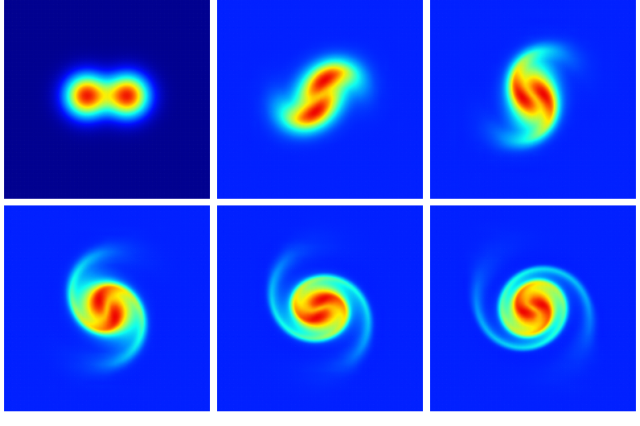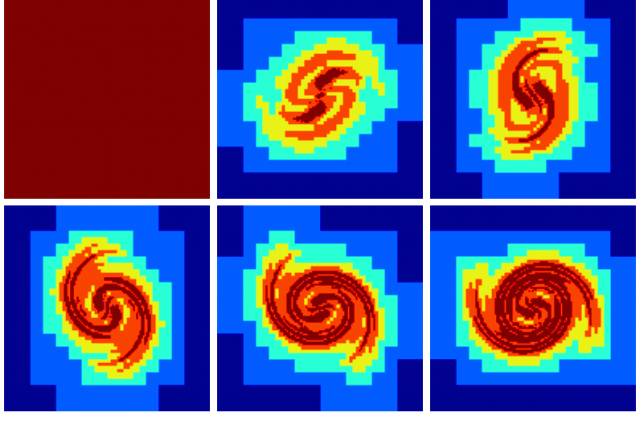# Merging of two vertices

Studying the interaction of two incompressible vortices is interesting for example as a basic model of two-dimensional turbulence. Here we solve the incompressible 2D Euler equations using a vorticity–streamfunction formulation.

#include "navier-stokes/stream.h"

The domain is centered on (0,0) and the maximum level of refinement is 8 i.e. the initial grid has N=2^8=256 grid points per dimension.

#define MAXLEVEL 8

int main()
{
origin (-0.5, -0.5);
init_grid (1 << MAXLEVEL);
run();
}

The initial vorticity field is composed of two Gaussian vortices separated by twice dd and with caracteristic radii dd/10.

event init (i = 0)
{
double dd = 0.1;
foreach()
omega[] = (exp(-(sq(x - dd) + sq(y))/(dd/10.)) +
exp(-(sq(x + dd) + sq(y))/(dd/10.)));
}

We output some statistics on the vorticity field and Poisson solver at the start and end of the simulation.

event logfile (t = {0,30}) {
stats s = statsf (omega);
fprintf (stderr, "%g %d %g %g %d\n", t, i, dt, s.sum, mgpsi.i);
}

We output the vorticity and level fields at regular intervals in a format compatible with gnuplot.

event output (t += 5) {
static int nf = 0;
char name;
sprintf (name, "omega-%d", nf);
FILE * fp = fopen (name, "w");
output_field ({omega}, fp, linear = true);
fclose (fp);

scalar l[];
foreach()
l[] = level;
sprintf (name, "level-%d", nf);
fp = fopen (name, "w");
output_field ({l}, fp);
fclose (fp);
nf++;
}

If we are using a quadtree grid, it is adapted using wavelet error control on \omega.

#if TREE
adapt_wavelet ({omega}, (double[]){1e-2}, MAXLEVEL, list = {omega, psi});
}
#endif

## Results

After running and processing by gnuplot we get:

set term @PNG enhanced size 640,426
set output 'vorticity.png'
set size ratio -1
unset key
unset xtics
unset ytics
unset border
unset colorbox
set pm3d
set pm3d map interpolate 1,1
set palette defined ( 0 0 0 0.5647, 0.125 0 0.05882 1, 0.25 0 0.5647 1, \
0.375 0.05882 1 0.9333, 0.5 0.5647 1 0.4392, \
0.625 1 0.9333 0, 0.75 1 0.4392 0, \
0.875 0.9333 0 0, 1 0.498 0 0 )

set multiplot layout 2,3 scale 1.6,1.6
splot 'omega-0'
splot 'omega-1'
splot 'omega-2'
splot 'omega-3'
splot 'omega-4'
splot 'omega-5'
unset multiplotEvolution of the vorticity field with time. (script)

set output 'level.png'
set cbrange [3:8]
set multiplot layout 2,3 scale 1.6,1.6
splot 'level-0'
splot 'level-1'
splot 'level-2'
splot 'level-3'
splot 'level-4'
splot 'level-5'
unset multiplotEvolution of level of refinement with time. (script)